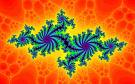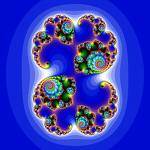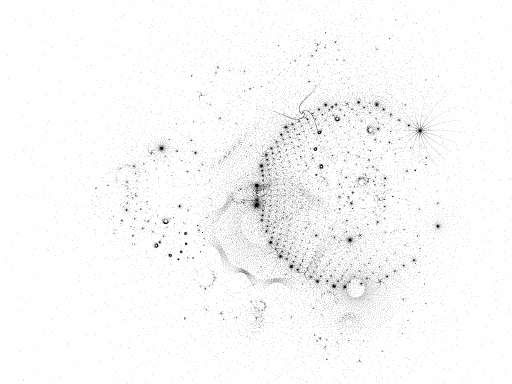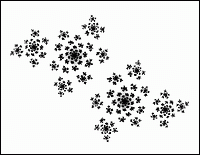# The Julia Set FractalsAside from the Mandelbrot set, the most famous fractals are the Julia sets. You’ve almost definitely seen images of the Julias (like the ones scattered through this post), but what you might not have realized is just how closely related the Julia sets are to the Mandelbrot set.

Remember what the mandelbrot set is? I’ll run through a very brief refresher, but if you want more details, you can look at my earlier post about it.Take a simple quadratic function in the complex plane: f(x)=x2+c, where c is a complex constant. If you iterate f, starting with f(0) – f(0), f2(0) = f(f(0)), f3(0)=f(f(f(0))), …, then depending on the value of c in the function f, either that series will always stay finite, or it will diverge to infinity.

So – from that, we can think about the family of functions, parameterized by C-values, where fk is the function x2+x. Using that family of functions, we can ask
for any given C, does the iteration series of fc(0) diverge? The Mandelbrot set is the set of c-values for which fc never diverges.So what’s a Julia set? Basically, it’s a kind of mirror image of the Mandelbrot. Take the same basic family of functions: f(x)=x2+c. But instead of varying
c, keep C fixed, and vary x. The Julia set for c is the set of x-values for which iterating f
does not diverge. There’s an infinite number of Julia sets – one for every possible C.If you work out the Julia sets for different C-values, you’ll find that there are some sets
that are connected, and some that aren’t. The non-connected ones are examples of what Mandelbrot called dusts – complex relatives of the Cantor dust; they’re generally called Fatou dusts. The connected Julia sets are often strikingly beautiful. Personally, I find some of the dusts even more beautiful than the connected ones.Knowing that the Julia sets are just a different parameterization of the same basic thing, you’d
expect there to be a deeper relationship between the sets. And you wouldn’t be disappointed. The Mandelbrot set is precisely the set of C-values for which the Julia set of fC is connected, and the Fatou dusts are the Julia sets of C-values that are not in the Mandelbrot set.

## 9 thoughts on “The Julia Set Fractals”

1.Xyz

It’s worth noting that the Julia sets were discovered by mathematician and WWI veteran Gaston Julia:
Biography

2.John Armstrong

Also, the miracle is that the Julia set for a given value of c is connected if and only if it contains the point z=0.

3.Token

“fk is the function x2+x”
Typo there?

4.Paul Carpenter

What does the image represent then? Is it points on an Argand diagram representing all the values of x? (real part of x being the horizontal axis and imaginary part being the vertical axis?)
Am I right that the colours represent how quickly the non-members of the set diverge?

5.Jonathan Vos Post

The theory goes deeper.
Today on arXiv:
http://arxiv.org/PS_cache/arxiv/pdf/0708/0708.3187v1.pdf
arXiv:0708.3187
Title: Dynamical properties and structure of Julia sets of postcritically bounded polynomial semigroups
Authors: Rich Stankewitz, Hiroki Sumi
Subjects: Dynamical Systems (math.DS); Complex Variables (math.CV)
We discuss the dynamic and structural properties of polynomial semigroups, a natural extension of iteration theory to random (walk) dynamics, where the semigroup G of complex polynomials (under the operation of composition of functions) is such that there exists a bounded set in the plane which contains any finite critical value of any map g in G. In general, the Julia set of such a semigroup G may be disconnected, and each Fatou component of such G is either simply connected or doubly connected. In this paper, we show that for any two distinct Fatou components of certain types (e.g., two doubly connected components of the Fatou set), the boundaries are separated by a Cantor set of quasicircles (with uniform dilatation) inside the Julia set of G. Important in this theory is the understanding of various situations which can and cannot occur with respect to how the Julia sets of the maps g in G are distributed within the Julia set of the entire semigroup G. We give several results in this direction and show how such results are used to generate (semi) hyperbolic semigroups possessing this postcritically boundedness condition.

6.Craig Helfgott

What I find interesting is that the Julia and Mandelbrot sets are two-dimensional slices of a larger 4-D set.
To construct the 4-D set, you start with the same map z->f(z) = z^2 + c, and this time allow both the initial value of z and the value of c to vary. Those points which remain within a bounded region are part of the 4-D set. The Mandelbrot set is what you obtain when you take the z_0 = 0 slice, and the Julia sets are what you obtain when you take constant c slices.
I don’t know what results there are on this larger set; I’m not terribly up on research in the field.

7.Xanthir, FCD

Paul Carpenter:
You are correct in all ways. Each point on the graph is a complex number, which is fed into the z -> z2+c function as the z_0 value. Black points converge, everything else diverges, and the colors are assigned based on how fast it diverges. You get the different Julia graphs by setting c to different values.
Exact same thing as the Mandelbrot, except there you set z_0 to be 0 and take each point as the value of c. There’s an entire family of Mandelbrots just like there is of Julias, we just don’t usually vary the z_0 term.
Craig Helfgott:
I wouldn’t mind seeing a projection or immersion or *something* of that set…

8.Ryan McGuire

Craig Helfgott, (more than five years later…)
Essentially, the usual Mandelbrot and Julia images that we’re all familiar with are specific instances of a more generalized equation / algorithm that map the 2D x and y coordinates of image pixels into the 4D space of [Zr, Zi, Cr, Ci]. For instance, for the standard Mandelbrot set image…
Zr = 0 = 0x + 0y + 0
Zi = 0 = 0x + 0y + 0
Cr = .01x + 0y – 2
Ci = 0x + .01y – 2

This maps x and y value in the range of [0,400] (an arguably reasonable size for an image) to C values with real and imaginary parts in the range [-2, +2].

That series of equations could be written in matrix form as…
[0 0 .01 0]
[Zr Zi Cr Ci] = [x y 1] * [0 0 0 .01]
[0 0 -2 -2]

(If those matrices come out skewed, the rows above and below the main part of the equation should be aligned with the last part on that middle row, making a 4×3 matrix.)

The transformation matrix for a (0, 0) Julia set would be…
[.01 0 0 0]
[0 .01 0 0]
[-2 -2 0 0]

We could take any number of different 2D slices through the 4D space just by creating a corresponding matrix. e.g. Try…
[.01 0 0 .01]
[0 .01 .01 0]
[-2 -2 -2 -2]

To be honest, I haven’t actually tried that. The four corners of a 400 x 400 image diverge, but (200, 200) doesn’t — there must be something interesting in between.